# Alpha blending and masking of images with Python, OpenCV, NumPy

Posted: 2019-05-14 / Tags: Python, OpenCV, NumPy, Image Processing

Performs alpha blending and masking with Python, OpenCV, NumPy.

It can be realized with only NumPy without using OpenCV. Because NumPy's array operation is easier and more flexible, I recommend it.

• Alpha blending with OpenCV: `cv2.addWeighted()`
• Masking with OpenCV: `cv2.bitwise_and()`
• Alpha blending with NumPy
• Complex alpha blending and masking with NumPy
• Mask image creation by OpenCV drawing

Refer to the following article about alpha blending and masking using Pillow (PIL).

The sample code uses the following image.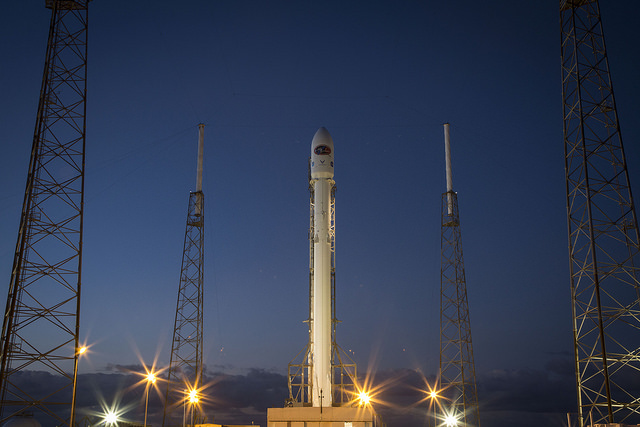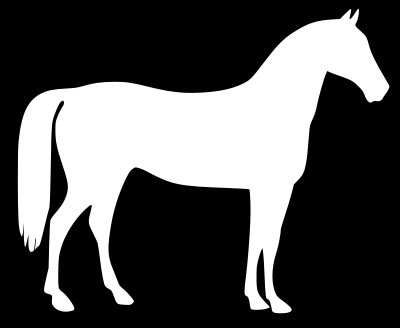OpenCV version of sample code is `4.0.1`. OpenCV3 and 4 should not change much, but OpenCV2 may be different, so be careful.

## Alpha blending with OpenCV: cv2.addWeighted()

Use `cv2.addWeighted()` to do alpha blending with OpenCV.

``````dst = cv2.addWeighted(src1, alpha, src2, beta, gamma[, dst[, dtype]])
``````

It is calculated as follows according to parameters.

``````dst = src1 * alpha + src2 * beta + gamma
``````

The two images need to be the same size, so resize them.

``````import cv2

src2 = cv2.resize(src2, src1.shape[1::-1])
``````

Refer to the following article for obtaining the size of the image read as NumPy array `ndarray`.

The image is alpha blended according to the values of the second parameter `alpha` and the fourth parameter `beta`.

Although images are saved as files here, if you want to display them in another window, you can use `cv2.imshow()` (eg: `cv2.imshow('window_name', dst)`). The same is true for the following sample code.

``````dst = cv2.addWeighted(src1, 0.5, src2, 0.5, 0)

``````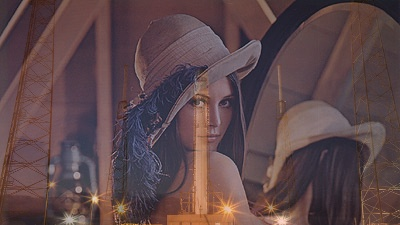The fifth parameter `gamma` is the value to be added to all pixel values.

``````dst = cv2.addWeighted(src1, 0.5, src2, 0.2, 128)

``````As you can see from the above result, it does not overflow even if it exceeds the maximum value (`255` for `uint8`), but it is noted that some data types may not be handled properly.

In such a case, use `clip()` method of `ndarray`. See the section on alpha blending with NumPy below.

Use `cv2.bitwise_and()` to do masking with OpenCV.

``````dst = cv2.bitwise_and(src1, src2[, dst[, mask]])
``````

`cv2.bitwise_and()` is a function that performs bitwise AND processing as the name suggests. The AND of the values for each pixel of the input images `src1` and `src2` is the pixel value of the output image.

Here, a grayscale image is used as a mask image for `src2`.

``````src2 = cv2.imread('data/src/horse_r.png')

src2 = cv2.resize(src2, src1.shape[1::-1])

print(src2.shape)
# (225, 400, 3)

print(src2.dtype)
# uint8

dst = cv2.bitwise_and(src1, src2)

cv2.imwrite('data/dst/opencv_bitwise_and.jpg', dst)
``````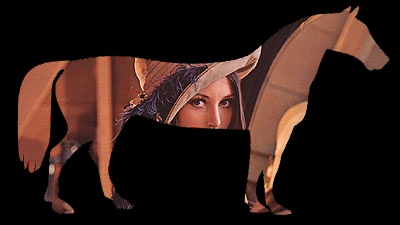When the image file is read, the data type is `uint8` (unsigned 8-bit integer: 0-255), black indicates pixel value `0` (`0b00000000` in binary), white indicates pixel value `255` (`0b11111111` in binary) ).

In the case of `uint8`, the result of the bit operation is easy to understand, but in the case of the floating point number `float`, it is noted that the bit operation is performed in binary notation and the result is unexpected.

It may be easier to understand the mask processing with NumPy described later.

In addition to `cv2.bitwise_and()`, OpenCV also includes `cv2.bitwise_or()`, `cv2.bitwise_xor()` and `cv2.bitwise_not()` for performing OR, XOR and NOT operation.

## Alpha blending with NumPy

Since NumPy can easily perform arithmetic operations for each pixels of the array, alpha blending can also be realized with a simple expression.

Here, image files are read as NumPy array `ndarray` using Pillow. Resize is also done by the method of Pillow.

Image files are read as ndarray with OpenCV's `cv2.imread()`, so it doesn't matter which OpenCV or Pillow is used, but be aware that the color order is different.

Since the operation of `ndarray` and scalar value is the operation of the value of each element and the scalar value, alpha blend can be calculated as follows. Be careful when saving as an image file with Pillow because the data type is cast automatically.

``````import numpy as np
from PIL import Image

src1 = np.array(Image.open('data/src/lena.jpg'))
src2 = np.array(Image.open('data/src/rocket.jpg').resize(src1.shape[1::-1], Image.BILINEAR))

print(src1.dtype)
# uint8

dst = src1 * 0.5 + src2 * 0.5

print(dst.dtype)
# float64

Image.fromarray(dst.astype(np.uint8)).save('data/dst/numpy_image_alpha_blend.jpg')
``````Note that when saving as a jpg file with the `save()` method of Pillow, you can specify the quality with the argument `quality` (it is omitted in the example, so it remains the default).

It is also easy if you want to add values to each pixel uniformly like the parameter `gamma` in OpenCV's `cv2.addWeighted()`. Different values can be added to each color as follows. As mentioned above, note that the color order differs depending on how the image file is read.

Use `clip()` to clip pixel values to the range `0` to `255`. Note that unexpected results occur when saving as an image file if there is a value exceeding the maximum value `255` of `uint8`.

``````dst = src1 * 0.5 + src2 * 0.2 + (96, 128, 160)

print(dst.max())
# 311.1

dst = dst.clip(0, 255)

print(dst.max())
# 255.0

Image.fromarray(dst.astype(np.uint8)).save('data/dst/numpy_image_alpha_blend_gamma.jpg')
``````Masking is easy with NumPy's array operations.

The arithmetic operations of arrays of the same `shape` are operations for each pixel at the same position.

The grayscale image read as `uint8` has `0` for black and `255` for white. By dividing this by `255`, black becomes `0.0` and white becomes `1.0`, and by multiplying this with the original image, only the white `1.0` part remains and the mask processing can be realized.

``````import numpy as np
from PIL import Image

src = np.array(Image.open('data/src/lena.jpg'))

# uint8 0 255

# float64 0.0 1.0

``````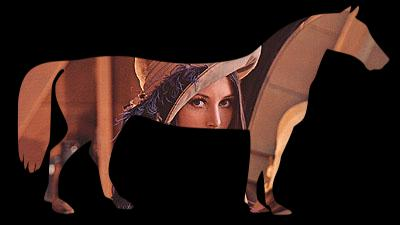In this example, if `dst = src * mask / 255`,`src * mask` is first calculated as `uint8` and the value is rounded and then divided by `255`, which is not the expected result.

It is OK if `dst = src * (mask / 255)` or `dst = mask / 255 * src`.

If you do not want to consider the order, you can cast all arrays to `float` and then perform the operation. There may be fewer mistakes.

Be careful if the mask image is a grayscale image and a 2D (no color dimension) `ndarray`. If multiplication is performed as it is, an error occurs.

``````mask = np.array(Image.open('data/src/horse_r.png').convert('L').resize(src.shape[1::-1], Image.BILINEAR))

# (225, 400)

# dst = src * mask
# ValueError: operands could not be broadcast together with shapes (225,400,3) (225,400)
``````

NumPy has a mechanism called broadcast that performs operations by automatically converting arrays of different dimensions and shapes as appropriate. However, according to the error message, broadcasting is not appropriately performed by the combination of the above examples.

It will broadcast well if you add one more dimension to a 2D `ndarray`.

``````mask = mask.reshape(*mask.shape, 1)

# (225, 400, 1)

``````

`shape` of the original array is unpacked and passed to `reshape()`.

Another way is to use `np.newaxis` instead of `reshape()`.

``````# mask = mask[:, :, np.newaxis]
``````

## Complex alpha blending and masking with NumPy

In the example of the alpha blend above, the image was composited at a uniform ratio over the entire surface of the image, but using NumPy, it is possible to composite based on another image (array).

Use the following gradation image. Gradation images can be generated using NumPy.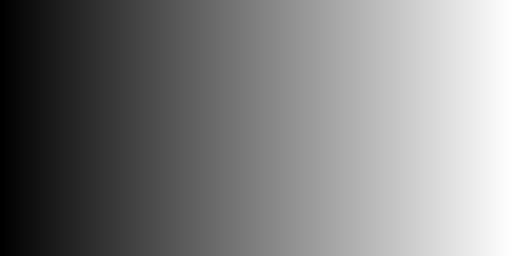It can be composited by a simple operation. An image is generated in which the alpha value (blending ratio) changes according to the pixel value of the gradation image.

``````import numpy as np
from PIL import Image

src1 = np.array(Image.open('data/src/lena.jpg'))
src2 = np.array(Image.open('data/src/rocket.jpg').resize(src1.shape[1::-1], Image.BILINEAR))

``````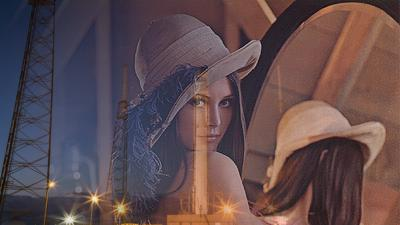It is also easy if you want to mask with another image.

``````mask2 = np.array(Image.open('data/src/horse_r.png').resize(src1.shape[1::-1], Image.BILINEAR))

``````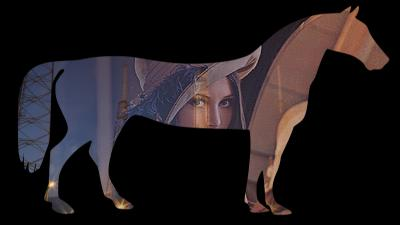## Mask image creation by OpenCV drawing

Geometric mask images can be created using the OpenCV drawing function.

It is possible to generate a `ndarray` of the same `shape` as the image to be processed by `np.zeros_like()` and in which all elements are `0`. It corresponds to a black image of the same size as the original image.

``````import cv2
import numpy as np

# (225, 400, 3)

# uint8
``````

You can also specify a size with `np.zeros()`.

Here, draw figures with the drawing function of OpenCV. A rectangle uses `cv2.rectangle()`, a circle uses `cv2.circle()`, and a polygon uses `cv2.fillConvexPoly()`. Rectangles and circles will be filled if `thickness=-1`.

``````cv2.rectangle(mask, (50, 50), (100, 200), (255, 255, 255), thickness=-1)
cv2.circle(mask, (200, 100), 50, (255, 255, 255), thickness=-1)
cv2.fillConvexPoly(mask, np.array([[330, 50], [300, 200], [360, 150]]), (255, 255, 255))

``````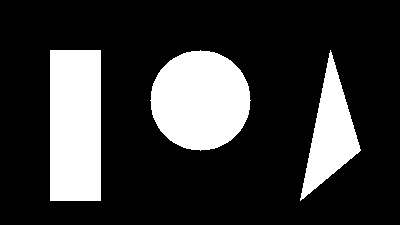When smoothing (blurring) processing is performed using a function such as `cv2.GaussianBlur()`, the boundary becomes smooth, so that it is possible to perform smooth synthesis by masking.

Specify the kernel size in the x direction and y direction as a tuple in the second parameter of `cv2.GaussianBlur()`. As each value is increased, the blurring width in that direction is increased. The value needs to be odd. The third parameter specifies the Gaussian standard deviation value. If it is `0`, it is calculated automatically. Note that it can not be omitted.

For other smoothing functions, refer to the official document below.

``````mask_blur = cv2.GaussianBlur(mask, (51, 51), 0)

````````````dst = src * (mask_blur / 255)

``````Note that if the part `dst = src * (mask_blur / 255)` is `dst = src * mask_blur / 255`, the result will not be as expected. See Masking with NumPy section.

Also, if the ndarray used as a mask is a two-dimensional array (no color dimension), it can not be calculated without adding one more dimension. See also Masking with NumPy section.